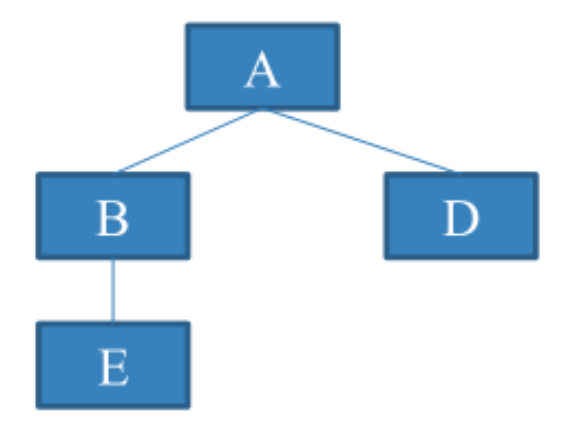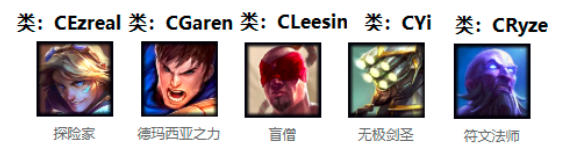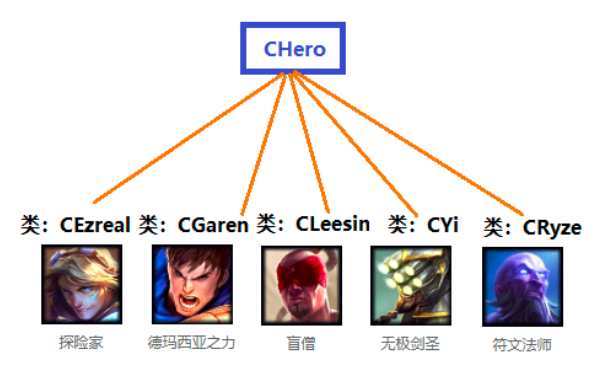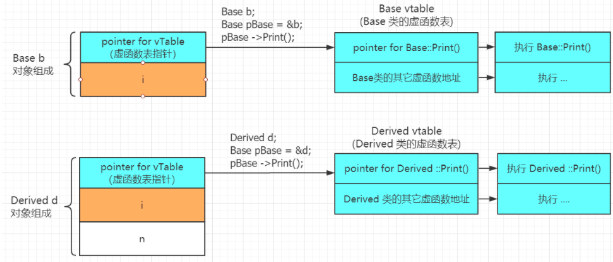# C++ 一篇搞懂多态的实现原理

0收藏

01 虚函数

virtual 关键字只用在类定义里的函数声明中，写函数体时不用。

``````class Base
{
virtual int Fun() ; // 虚函数
};

int Base::Fun() // virtual 字段不用在函数体时定义
{ }``````

02 多态的表现形式一

• 「派生类的指针」可以赋给「基类指针」；
• 通过基类指针调用基类和派生类中的同名「虚函数」时:
1. 若该指针指向一个基类的对象，那么被调用是基类的虚函数；

2. 若该指针指向一

``````// 基类
class CFather
{
public:
virtual void Fun() { } // 虚函数
};

// 派生类
class CSon : public CFather
{
public :
virtual void Fun() { }
};

int main()
{
CSon son;
CFather *p = &son;
p->Fun(); //调用哪个虚函数取决于 p 指向哪种类型的对象
return 0;
}``````

03 多态的表现形式二

• 派生类的对象可以赋给基类「引用」
• 通过基类引用调用基类和派生类中的同名「虚函数」时:
1. 若该引用引用的是一个基类的对象，那么被调

2. 若该引用引用的是一个派生类的对象，那么被

``````// 基类
class CFather
{
public:
virtual void Fun() { } // 虚函数
};

// 派生类
class CSon : public CFather
{
public :
virtual void Fun() { }
};

int main()
{
CSon son;
CFather &r = son;
r.Fun(); //调用哪个虚函数取决于 r 引用哪种类型的对象
return 0;
}
}``````

04 多态的简单示例

``````class A
{
public :
virtual void Print() { cout << "A::Print"<<endl ; }
};

// 继承A类
class B: public A
{
public :
virtual void Print() { cout << "B::Print" <<endl; }
};

// 继承A类
class D: public A
{
public:
virtual void Print() { cout << "D::Print" << endl ; }
};

// 继承B类
class E: public B
{
virtual void Print() { cout << "E::Print" << endl ; }
};``````

A类、B类、E类、D类的关系如下图：``````int main()
{
A a; B b; E e; D d;

A * pa = &a;
B * pb = &b;
D * pd = &d;
E * pe = &e;

pa->Print();  // a.Print()被调用，输出：A::Print

pa = pb;
pa -> Print(); // b.Print()被调用，输出：B::Print

pa = pd;
pa -> Print(); // d.Print()被调用，输出：D::Print

pa = pe;
pa -> Print(); // e.Print()被调用，输出：E::Print

return 0;
}``````

05 多态作用

LOL 英雄联盟游戏例子

LOL 英雄联盟是 5v5 竞技游戏，游戏中有很多英雄，每种英雄都有一个「类」与之对应，每个英雄就是一个「对象」。1.为每个英雄类编写 Attack、FightBack 和 Hurted 成员函数。
Attack 函数表示攻击动作；
FightBack 函数表示反击动作；
Hurted 函数表示减少自身生命值，并表现受伤动作。

2.设置基类CHero，每个英雄类都继承此基类02 非多态的实现方法

``````// 基类
class CHero
{
protected:
int m_nPower ; //代表攻击力
int m_nLifeValue ; //代表生命值
};

// 无极剑圣类
class CYi : public CHero
{
public:
// 攻击盖伦的攻击函数
void Attack(CGaren * pGaren)
{
.... // 表现攻击动作的代码
pGaren->Hurted(m_nPower);
pGaren->FightBack(this);
}

// 攻击瑞兹的攻击函数
void Attack(CRyze * pRyze)
{
.... // 表现攻击动作的代码
pRyze->Hurted(m_nPower);
pRyze->FightBack( this);
}

// 减少自身生命值
void Hurted(int nPower)
{
... // 表现受伤动作的代码
m_nLifeValue -= nPower;
}

// 反击盖伦的反击函数
void FightBack(CGaren * pGaren)
{
...．// 表现反击动作的代码
pGaren->Hurted(m_nPower/2);
}

// 反击瑞兹的反击函数
void FightBack(CRyze * pRyze)
{
...．// 表现反击动作的代码
pRyze->Hurted(m_nPower/2);
}
};``````

``````void Attack(CAshe * pAshe);
void FightBack(CAshe * pAshe);``````

03 多态的实现方式

``````// 基类
class CHero
{
public:
virtual void Attack(CHero *pHero){}
virtual voidFightBack(CHero *pHero){}
virtual void Hurted(int nPower){}

protected:
int m_nPower ; //代表攻击力
int m_nLifeValue ; //代表生命值
};

// 派生类 CYi:
class CYi : public CHero {
public:
// 攻击函数
void Attack(CHero * pHero)
{
.... // 表现攻击动作的代码
pHero->Hurted(m_nPower); // 多态
pHero->FightBack(this);  // 多态
}

// 减少自身生命值
void Hurted(int nPower)
{
... // 表现受伤动作的代码
m_nLifeValue -= nPower;
}

// 反击函数
void FightBack(CHero * pHero)
{
...．// 表现反击动作的代码
pHero->Hurted(m_nPower/2); // 多态
}
};``````

``````void Attack( CAshe * pAshe) ;
void FightBack(CAshe * pAshe) ;``````

``````void CYi::Attack(CHero * pHero)
{
pHero->Hurted(m_nPower); // 多态
pHero->FightBack(this);  // 多态
}

CYi yi;
CGaren garen;
CLeesin leesin;
CEzreal ezreal;

yi.Attack( &garen );  //(1)
yi.Attack( &leesin ); //(2)
yi.Attack( &ezreal ); //(3)``````

，分别调用：

``````CGaren::Hurted
CLeesin::Hurted
CEzreal::Hurted``````

``````class Base
{
public:
void fun1()
{
fun2();
}

virtual void fun2()  // 虚函数
{
cout << "Base::fun2()" << endl;
}
};

class Derived : public Base
{
public:
virtual void fun2()  // 虚函数
{
cout << "Derived:fun2()" << endl;
}
};

int main()
{
Derived d;
Base * pBase = & d;
pBase->fun1();
return 0;
}``````

``````class Base
{
public:
void fun1()
{
this->fun2();  // this是基类指针，fun2是虚函数，所以是多态
}
}``````

this 指针的作用就是指向成员函数所作用的对象， 所以非静态成员函数中可以直接使用 this 来代表指向该函数作用的对象的指针。

pBase 指针对象指向的是派生类对象，派生类里没有 fun1 成员函数，所以就会调用基类的 fun1 成员函数，在Base::fun1() 成员函数体里执行 this->fun2() 时，实际上指向的是派生类对象的 fun2 成员函数。

所以正确的输出结果是：

``Derived:fun2()``

``````// 基类
class CFather
{
public:
virtual void hello() // 虚函数
{
cout<<"hello from father"<<endl;
}

virtual void bye() // 虚函数
{
cout<<"bye from father"<<endl;
}
};

// 派生类
class CSon : public CFather
{
public:
CSon() // 构造函数
{
hello();
}

~CSon()  // 析构函数
{
bye();
}

virtual void hello() // 虚函数
{
cout<<"hello from son"<<endl;
}
};

int main()
{
CSon son;
CFather *pfather;
pfather = & son;
pfather->hello(); //多态
return 0;
}``````

``````hello from son  // 构造son对象时执行的构造函数
hello from son  // 多态
bye from father // son对象析构时，由于CSon类没有bye成员函数，所以调用了基类的bye成员函数``````

「多态」的关键在于通过基类指针或引用调用一个虚函数时，编译时不能确定到底调用的是基类还是派生类的函数，运行时才能确定。

``````class A
{
public:
int i;
virtual void Print() { } // 虚函数
};

class B
{
public:
int n;
void Print() { }
};

int main()
{
cout << sizeof(A) << ","<< sizeof(B);
return 0;
}``````

``16,4``

01 虚函数表

``````// 基类
class Base
{
public:
int i;
virtual void Print() { } // 虚函数
};

// 派生类
class Derived : public Base
{
public:
int n;
virtual void Print() { } // 虚函数
};``````02 证明虚函数表指针的作用

``````// 基类
class A
{
public:
virtual void Func()  // 虚函数
{
cout << "A::Func" << endl;
}
};

// 派生类
class B : public A
{
public:
virtual void Func()  // 虚函数
{
cout << "B::Func" << endl;
}
};

int main()
{
A a;

A * pa = new B();
pa->Func(); // 多态

// 64位程序指针为8字节
int * p1 = (int *) & a;
int * p2 = (int *) pa;

* p2 = * p1;
pa->Func();

return 0;
}``````

``````B::Func
A::Func``````
• 第 25-26 行代码中的 pa 指针指向的是 B 类对象，所以 pa->Func() 调用的是 B 类对象的虚函数 Func()，输出内容是 B::Func ；
• 第 29-30 行代码的目的是把 A 类的头 8 个字节的「虚函数表指针」存放到 p1 指针和把 B 类的头 8 个字节的「虚函数表指针」存放到 p2 指针；
• 第 32 行代码目的是把 A 类的「虚函数表指针」 赋值给 B 类的「虚函数表指针」，所以相当于把 B 类的「虚函数表指针」 替换 成了 A 类的「虚函数表指针」；
• 由于第 32 行的作用，把 B 类的「虚函数表指针」 替换 成了 A 类的「虚函数表指针」，所以第 33 行调用的是 A 类的虚函数 Func()，输出内容是 A::Func

``````// 基类
class A
{
public:
A()  // 构造函数
{
cout << "construct A" << endl;
}

~A() // 析构函数
{
cout << "Destructor A" << endl;
}
};

// 派生类
class B : public A
{
public:
B()  // 构造函数
{
cout << "construct B" << endl;
}

~B()// 析构函数
{
cout << "Destructor B" << endl;
}
};

int main()
{
A *pa = new B();
delete pa;

return 0;
}``````

``````construct A
construct B
Destructor A``````

• 派生类的析构函数可以 virtual 不进行声明；
• 通过基类的指针删除派生类对象时，首先调用派生类的析构函数，然后调用基类的析构函数，还是遵循「先构造，后虚构」的规则。

``````// 基类
class A
{
public:
A()
{
cout << "construct A" << endl;
}

virtual ~A() // 虚析构函数
{
cout << "Destructor A" << endl;
}
};``````

``````construct A
construct B
Destructor B
Destructor A``````

• 一个类如果定义了虚函数，则应该将析构函数也定义成虚函数;
• 或者，一个类打算作为基类使用，也应该将析构函数定义成虚函数。
• 注意：不允许构造函数不能定义成虚构造函数。

``````class A
{

public:
virtual void Print( ) = 0 ; //纯虚函数
private:
int a;
};``````

• 抽象类只能作为基类来派生新类使用，不能创建抽象类的对象
• 抽象类的指针和引用可以指向由抽象类派生出来的类的对象

``````A a;         // 错，A 是抽象类，不能创建对象
A * pa ;     // ok,可以定义抽象类的指针和引用
pa = new A ; // 错误, A 是抽象类，不能创建对象``````帖子
视频
声望
粉丝
社区精华内容